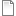# Individual-based model of population dynamics with movement of individual animals

ModelId:
animal1
SimileVersion:
5.9

This models the dynamics of a population of animals, represented as a set of individuals that come into existence, live for a while, then die. When the animals are alive, they move around according to a simple random walk.Equations:

Equations in Desktop

N = sum({one})

Equations in ANIMAL
X: initial value = rand(30,70)
Y: initial value = rand(30,70)
Dir: initial value = rand(0,6.28)

create = 100
r = rand(0,2*0.7)
m = 0.6

xchange = (if outside == 1 then speed*cos(dir+3.14) else speed*cos(dir))
dirchange = (if outside == 1 then 3.14/dt(1) else rand(-0.4,0.4)/dt(1))
ychange = (if outside == 1 then speed*sin(dir+3.14) else speed*sin(dir))

speed = 5
size = 3
outside = (if (x<0;x>100;y<0;y>100)then 1 else 0)
one = 1

Results:AttachmentSizeanimal1.sml95.71 KBanimal1.shf2.1 KB
Model tags: